Next: References Up: Incompressible Euler equations Previous: The vorticity formulation

## The velocity formulation

Following  our goal is introduce a second-order central difference scheme for incompressible flows, based on velocity variables. The use of the velocity formulation yields a more versatile algorithm. The advantage of our proposed central scheme in its velocity formulation is two-fold: generalization to the three dimensional case is straightforward, and the treatment of boundary conditions associated with general geometries becomes simpler. The result is a simple fast high-resolution method, whose accuracy is comparable to that of an upwind scheme. In addition, numerical experiments show the new scheme to be immune to some of the well-known deleterious consequences of under-resolution.

We consider a two-dimensional incompressible flow field,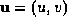, so that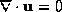. The equations of motion for a Newtonian fluid in conservation form are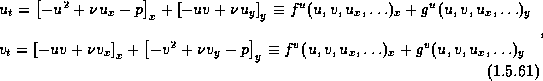where p is the pressure,is the kinematic viscosity, and subscripts denote partial derivatives. The functions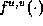and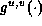are components of the fluxes of the conserved quantities u and v.

The computational grid consists of rectangular cells of sizes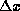and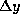; at time level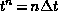, these cells,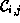, are centered at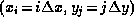. Starting with the corresponding cell averages,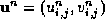, we first reconstruct a piecewise linear polynomial approximation which recovers the point values of the velocity field,. For second-order accuracy, the piecewise linear reconstructed velocities take the form,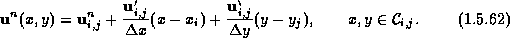As before, exact averaging over a staggered control volume yieldsand a similar averaging applies for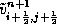.

An exact computation yields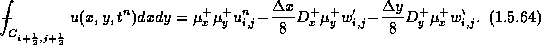The incompressible fluxes, e.g.,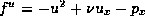, are approximated in terms of the midpoint rule , which in turn employs predicted midvalues which are obtained from half-step Taylor expansion. Thus our scheme starts with a predictor step of the form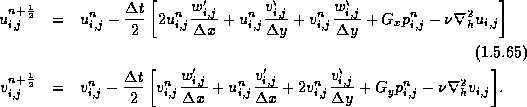Note that the predictor step is nothing but a forward Euler scheme; conservation form is not essential for the spatial discretization at this stage.

This is followed by a corrector step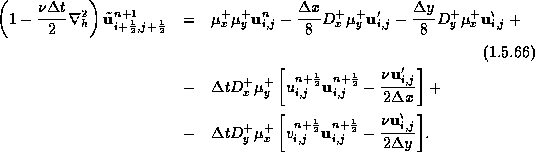Note that the viscous terms are handled here by the implicit Crank-Nicholson discretization which is favored due to its preferable stability properties. Here, we ignore the pressure terms; instead, the contribution of the pressure will be integrated by enforcing zero-divergence fluxes at the last projection step.

Compute the potential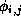solving the Poisson equation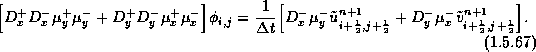Then, the pressure gradient at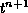is being updated,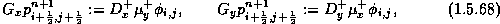and finally, it is used to evaluate the divergence-free velocity field,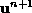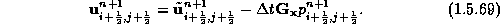In Figure 1.5.15, we plot vorticity contours for two shear layer problems studied in : the inviscid ``thick'' shear layer problem corresponding to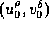with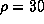, and a viscous ``thin'' shear layer problem (with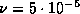), corresponding towith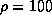. As in , both plots in Figures 1.5.15a and 1.5.15b are recorded at time t=1.2, and are subject to an initial perturbation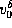, with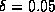.
Further applications of the central schemes for more complex incompressible flows (with 'variable' axisymmetric coefficients, forcing source/viscous terms, ...), can be found in ,.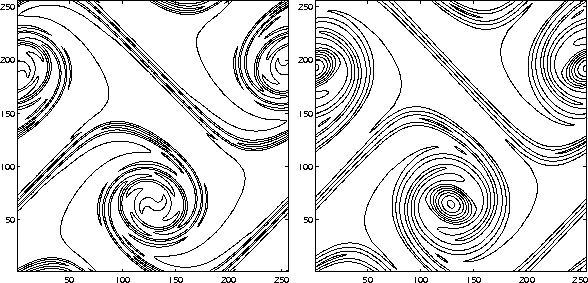Figure 1.5.15: Contour lines of the vorticity,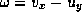, at t=1.2 with initial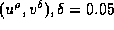, using a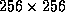grid. (a) A ``thick'' shear layer with, and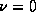. The contour levels range from -36 to 36 (cf. Figure 3c in Ref. ). (b) A ``thin'' shear layer with, and. The contour levels range from -70 to 70 (cf. Figure 9b in Ref. ).Next: References Up: Incompressible Euler equations Previous: The vorticity formulation